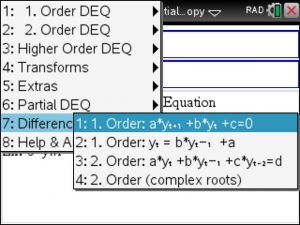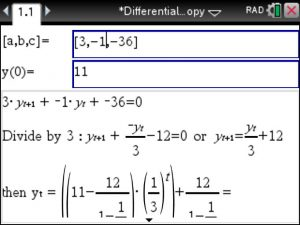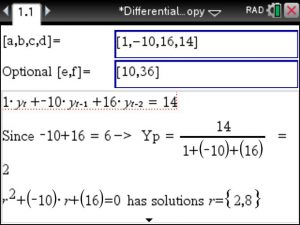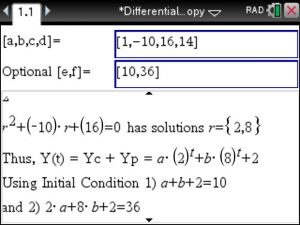## ▷Difference Equations – Step by Step – using the TiNSpire CX CAS : Differential Equations Made Easy

Solving Difference Equations using a TiNspire CX CAS can be done using the Differential Equations Made Easy app as shown below :

Select either 1. or 2. order Difference Equations:Say, we start with a 1. order Difference Equation, we enter the coefficients a , b and c and the initial value y(0) as shown in image below:Plugging in the given values into the general solution yields:Lets also take a look at a 2. order Difference Equation:How nice is this? Solutions to any 1. and 2. order Difference Equations can be found using the handy TiNspire CX CAS , and using the Differential Equations Made Easy app at www.tinspireapps.com

Posted on Categories difference equation